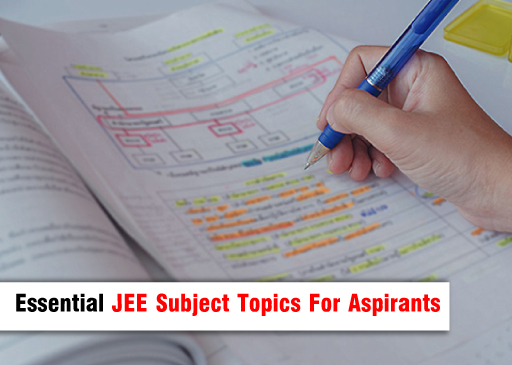# Essential JEE Subject Topics For AspirantsMon Mar 20, 2023
|
Gursharan Singh

The JEE is regarded as one of the most difficult and competitive examinations in the world and a crucial exam in India. To pass this test, you must put in a lot of effort, devotion, and planning.

Students must concentrate on a few key areas in order to succeed in JEE and one must start preparing these topics from 11th grade. These themes in this blog by Momentum, an institute for having the best JEE Main Coaching in Gorakhpur, are critical to laying a solid basis for the test and ensuring long-term success.

### Physics:

• Mechanics (Newton's laws, Work, Energy, Power, Friction, Circular Motion, and so on)
• Thermal Physics (Thermal expansion, Heat transfer, Thermodynamics, etc.)
• Waves (Simple Harmonic Motion, Sound Waves, etc)
• Optics (Reflection & Refraction, Dispersion, etc)
• Electricity and magnetism (Electric field and Potential, Gauss' Law, capacitance, current electricity, magnetic field and force, and so on)
• Modern Physics (Atomic Structure, Nuclear Physics, etc.)

### Chemistry:

• Chemistry Fundamental Concepts (Atoms, Molecules, Chemical Equations, etc.)
• Matter's States (Gases, Liquids, and Solids)
• Chemical Thermodynamics (Thermochemistry, Entropy, etc.)
• Chemical Equilibrium (Acid-Base Equilibria, Redox Reactions, etc.)
• Atomic Structure (Bohr's Atomic Model, Quantum Mechanics, and so on)
• Chemical Bonding and Molecular Structure (VSEPR Theory, Hybridization, etc.)
• Chemical Kinetics (Rate of Reactions, Activation Energy, etc.)

### Maths:

• Algebra (Quadratic Equations, Progressions, Logarithms, etc)
• Trigonometry (Trigonometric Ratios, Identities, etc)
• Coordinate Geometry (Lines, Circles, Conic Sections, etc.)
• Calculus (Limits and Continuity, Derivatives, Integrals, etc)
• Vectors and 3D Geometry (Vector Algebra, Scalar Triple Product, etc.)
• Probability (Permutations and Combinations, Conditional Probability, etc)

Please keep in mind that these are just some of the major themes; you should also concentrate on the fundamentals and principles of each subject. Also, the curriculum may differ somewhat depending on the board or school system you choose.

Here is an Elaboration on some topics for 11th-class students:

### Calculus:

It is one of the most significant subjects for IIT JEE and can be found in 11th grade. It comprises differential and integral calculus, which are essential for comprehending fundamental calculus ideas. Limits, continuity, differentiation, and integration are the building elements of calculus. If you want to have an excellent score in the JEE, you can prefer to have the most excellent JEE Advanced Coaching in Gorakhpur. Here you get decent coaching from highly educated mentors.

### Algebra

Algebra is another key subject for IIT JEE in 11th grade. It covers subjects like linear equations, quadratic equations, matrices, and determinants. Students should concentrate on solving problems using algebraic expressions, equations, and inequalities.

### Trigonometry

Trigonometry is another important subject for IIT JEE in 11th grade. It covers trigonometric functions, identities, and equations. Students should concentrate on knowing the fundamental ideas of trigonometry and how to use them to solve problems.

### Mechanics

Mechanics is a key subject for IIT JEE in 11th grade. It covers subjects such as kinematics, rules of motion, work, energy, and power. Students should concentrate on knowing the fundamental ideas of mechanics and how to use them in problem-solving.